﻿ 最新十种深度学习算法要点及代码解析 - 挽手话别语# 最新十种深度学习算法要点及代码解析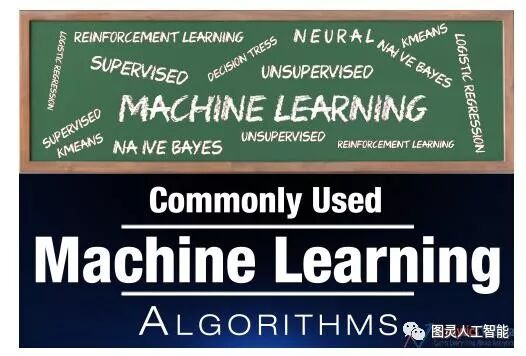1、 监督式学习

2、非监督式学习

3、强化学习

1. 线性回归

2. 逻辑回归

3. 决策树

4. SVM

5. 朴素贝叶斯

6. K最近邻算法

7. K均值算法

8. 随机森林算法

9. 降维算法

1、线性回归

• Y：因变量

• a：斜率

• x：自变量

• b ：截距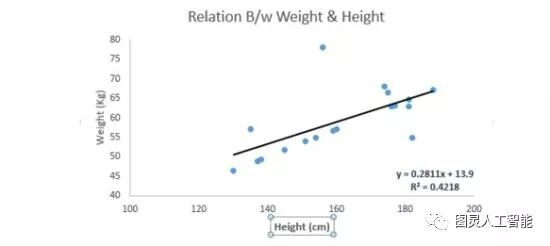Python 代码

`#Import Library#Import other necessary libraries like pandas, numpy...from sklearn import linear_model #Load Train and Test datasets#Identify feature and response variable(s) and values must be numeric and numpy arraysx_train=input_variables_values_training_datasetsy_train=target_variables_values_training_datasetsx_test=input_variables_values_test_datasets # Create linear regression objectlinear = linear_model.LinearRegression() # Train the model using the training sets and check scorelinear.fit(x_train, y_train)linear.score(x_train, y_train) #Equation coefficient and Interceptprint('Coefficient: n', linear.coef_)print('Intercept: n', linear.intercept_) #Predict Outputpredicted= linear.predict(x_test)`

R代码

`#Load Train and Test datasets#Identify feature and response variable(s) and values must be numeric and numpy arraysx_train <- input_variables_values_training_datasetsy_train <- target_variables_values_training_datasetsx_test <- input_variables_values_test_datasetsx <- cbind(x_train,y_train)# Train the model using the training sets and check scorelinear <- lm(y_train ~ ., data = x)summary(linear)#Predict Outputpredicted= predict(linear,x_test)`

2、逻辑回归

`odds= p/ (1-p) = probability of event occurrence / probability of not event occurrenceln(odds) = ln(p/(1-p))logit(p) = ln(p/(1-p)) = b0+b1X1+b2X2+b3X3....+bkXk`Python代码

`#Import Libraryfrom sklearn.linear_model import LogisticRegression#Assumed you have, X (predictor) and Y (target) for training data set and x_test(predictor) of test_dataset# Create logistic regression objectmodel = LogisticRegression() # Train the model using the training sets and check scoremodel.fit(X, y)model.score(X, y) #Equation coefficient and Interceptprint('Coefficient: n', model.coef_)print('Intercept: n', model.intercept_) #Predict Outputpredicted= model.predict(x_test)`

R代码

`x <- cbind(x_train,y_train)# Train the model using the training sets and check scorelogistic <- glm(y_train ~ ., data = x,family='binomial')summary(logistic)#Predict Outputpredicted= predict(logistic,x_test)`

• 加入交互项

• 精简模型特性

• 使用正则化方法

• 使用非线性模型

3、决策树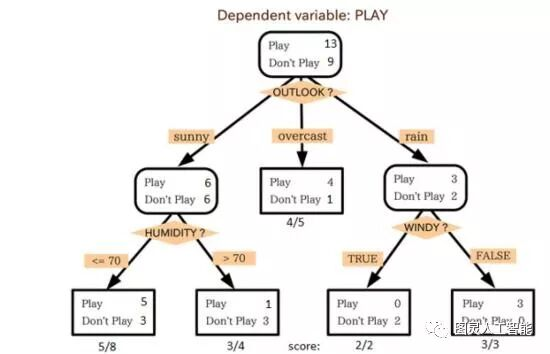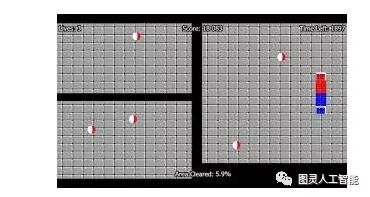Python代码

`#Import Library#Import other necessary libraries like pandas, numpy...from sklearn import tree #Assumed you have, X (predictor) and Y (target) for training data set and x_test(predictor) of test_dataset# Create tree object model = tree.DecisionTreeClassifier(criterion='gini') # for classification, here you can change the algorithm as gini or entropy (information gain) by default it is gini  # model = tree.DecisionTreeRegressor() for regression# Train the model using the training sets and check scoremodel.fit(X, y)model.score(X, y) #Predict Outputpredicted= model.predict(x_test)`

R代码

`library(rpart)x <- cbind(x_train,y_train)# grow tree fit <- rpart(y_train ~ ., data = x,method="class")summary(fit)#Predict Output predicted= predict(fit,x_test)`

4、支持向量机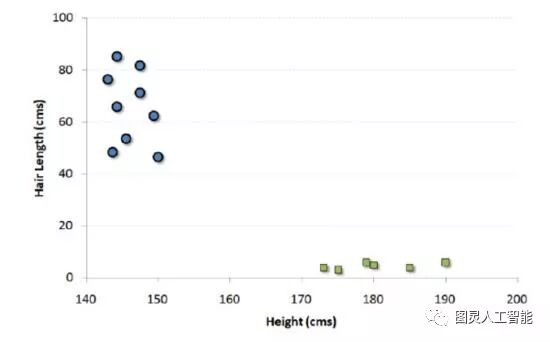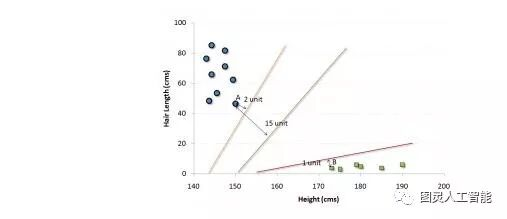• 比起之前只能在水平方向或者竖直方向画直线，现在你可以在任意角度画线或平面。

• 游戏的目的变成把不同颜色的球分割在不同的空间里。

• 球的位置不会改变。

Python代码

`#Import Libraryfrom sklearn import svm#Assumed you have, X (predictor) and Y (target) for training data set and x_test(predictor) of test_dataset# Create SVM classification object model = svm.svc() # there is various option associated with it, this is simple for classification. You can refer link, for mo# re detail.# Train the model using the training sets and check scoremodel.fit(X, y)model.score(X, y)#Predict Outputpredicted= model.predict(x_test)`

R代码

`library(e1071)x <- cbind(x_train,y_train)# Fitting modelfit <-svm(y_train ~ ., data = x)summary(fit)#Predict Output predicted= predict(fit,x_test)`

5、朴素贝叶斯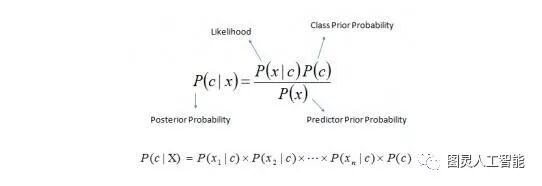• P ( c|x ) 是已知预示变量（属性）的前提下，类（目标）的后验概率

• P ( c ) 是类的先验概率

• P ( x|c ) 是可能性，即已知类的前提下，预示变量的概率

• P ( x ) 是预示变量的先验概率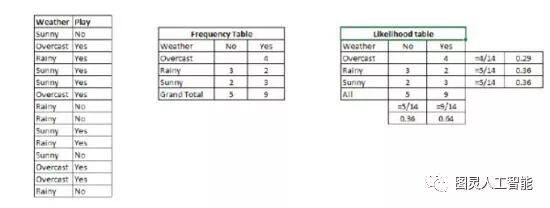Python代码

```#Import Libraryfrom sklearn.naive_bayes import GaussianNB #Assumed you have, X (predictor) and Y (target) for training data set and x_test(predictor) of test_dataset# Create SVM classification object model = GaussianNB() # there is other distribution for multinomial classes like Bernoulli Naive Bayes, Refer link# Train the model using the training sets and check score

```